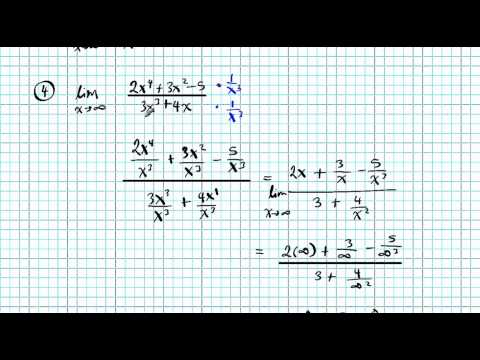# maths gotserved► Play all

# Limits

Learn how to evaluate limits with different techniques
The student will define and apply the properties of elementary functions, including
algebraic, trigonometric, exponential, and composite functions and their inverses, and
graph these functions, using a graphing calculator. Properties of functions will include
domains, ranges, combinations, odd, even, periodicity, symmetry, asymptotes, zeros,
upper and lower bounds, and intervals where the function is increasing or decreasing.
APC.2 The student will define and apply the properties of limits of functions. Limits will be
evaluated graphically and algebraically. This will include
a) limits of a constant;
b) limits of a sum, product, and quotient;
c) one-sided limits; and
d) limits at infinity, infinite limits, and non-existent limits. *
*AP Calculus BC will include l'Hopital's Rule, which will be used to find the limit of
functions whose limits yield the indeterminate forms: 0/0 and ∞/∞.
APC.3 The student will use limits to define continuity and determine where a function is
continuous or discontinuous. This will include
a) continuity in terms of limits;
b) continuity at a point and over a closed interval;
c) application of the Intermediate Value Theorem and the Extreme Value Theorem; and
d) geometric understanding and interpretation of continuity and discontinuity.
APC.4 The student will investigate asymptotic and unbounded behavior in functions. This will
include
a) describing and understanding asymptotes in terms of graphical behavior and limits
involving infinity; and
b) comparing relative magnitudes of functions and their rates of change.
APC.5 The student will investigate derivatives presented in graphic, numerical, and analytic
contexts and the relationship between continuity and differentiability. The derivative will
be defined as the limit of the difference quotient and interpreted as an instantaneous rate
of change.
Mathematics Standards of Learning
60
APC.6 The student will investigate the derivative at a point on a curve. This will include
a) finding the slope of a curve at a point, including points at which the tangent is vertical
and points at which there are no tangents;
b) using local linear approximation to find the slope of a tangent line to a curve at the
point;
c) defining instantaneous rate of change as the limit of average rate of change; and
d) approximating rate of change from graphs and tables of values.
APC.7 The student will analyze the derivative of a function as a function in itself. This will
include
a) comparing corresponding characteristics of the graphs of f, f ', and f ";
b) defining the relationship between the increasing and decreasing behavior of f and the
sign of f ';
Questions,lecture,math,your,placement,free,ideas,trick,intermediate value theorem,help,continuity,tutor,self-help
c) translating verbal descriptions into equations involving derivatives and vice versa;
d) analyzing the geometric consequences of the Mean Value Theorem;
e) defining the relationship between the concavity of f and the sign of f "; and
f) identifying points of inflection as places where concavity changes and finding points
of inflection.
APC.8 The student will apply the derivative to solve problems. This will include
a) analysis of curves and the ideas of concavity and monotonicity;
b) optimization involving global and local extrema;
c) modeling of rates of change and related rates;
d) use of implicit differentiation to find the derivative of an inverse function;
e) interpretation of the derivative as a rate of change in applied contexts, including
velocity, speed, and acceleration; and
f) differentiation of nonlogarithmic functions, using the technique of logarithmic
differentiation. *
* AP Calculus BC will also apply the derivative to solve problems. This will include
a) analysis of planar curves given in parametric form, polar form, and vector form,
including velocity and acceleration vectors;
b) numerical solution of differential equations, using Euler's method;
c) l'Hopital's Rule to test the convergence of improper integrals and series; and
d) geometric interpretation of differential equations via slope fields and the relationship
between slope fields and the solution curves for the differential equations.
APC.9 The student will apply formulas to find derivatives. This will include
a) derivatives of algebraic, trigonometric, exponential, logarithmic, and inverse
trigonometric functions;
b) derivations of sums, products, quotients, inverses, and composites (chain rule) of
elementary functions;
c) derivatives of implicitly defined functions; and
d) higher order derivatives of algebraic, trigonometric, exponential, and logarithmic,
functions. *
* AP Calculus BC will also include finding derivatives of parametric, polar, and vector
functions.
Mathematics Standards of Learning
61
APC.10 The student will use Riemann sums and the Trapezoidal Rule to approximate definite
Learn how to evaluate limits with different techniques
The student will define and apply the properties of elementary functions, including
algebraic, trigonometric, exponential, and composite functions and their inverses, and
graph these functions...